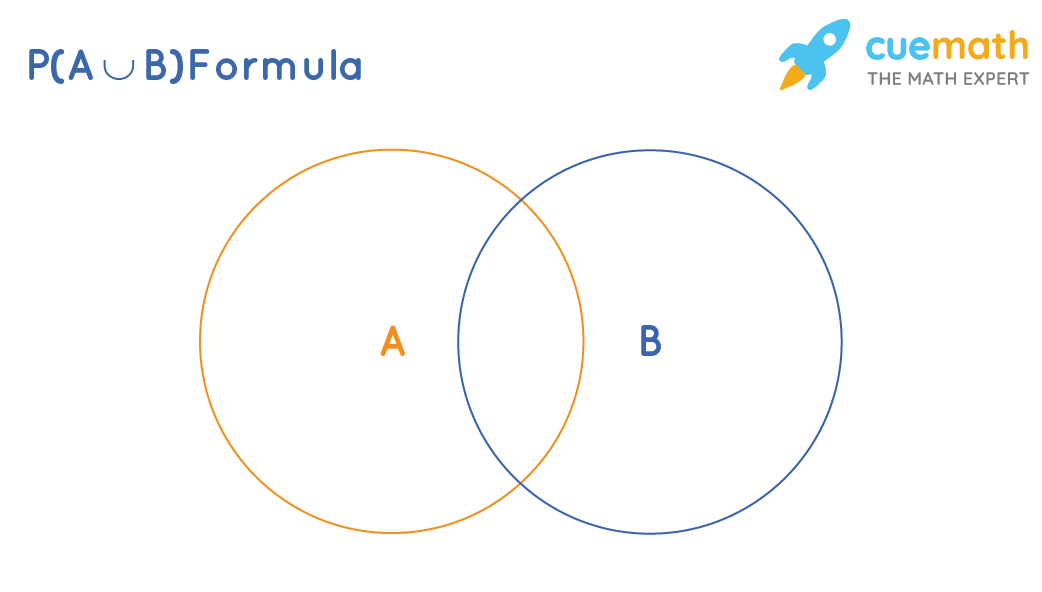# P(A∪B) Formula

The symbol "∪" (union) means "or". i.e., P(A∪B) is the probability of happening of the event A or B. To find, P(A∪B), we have to count the sample points that are present in both A and B. So is P(A∪B) = P(A) + P(B)? No, because while counting the sample points from A and B, the sample points that are in A∩B are counted twice. Thus, we need to subtract P(A∩B) from the above sum to get P(A∪B).P(A∪B) = P(A) + P(B) - P(A∩B)

## What is P(A∪B) Formula ?

From the above explanation, the P(A∪B) formula is:

P(A∪B) = P(A) + P(B) - P(A∩B)

This is also known as the addition theorem of probability.

But what if events A and B are mutually exclusive? In that case, P(A∩B) = 0.

The P(A∪B) formula when A and B are mutually exclusive is,

P(A∪B) = P(A) + P(B)Have questions on basic mathematical concepts?
Become a problem-solving champ using logic, not rules. Learn the why behind math with our certified experts

Book a Free Trial Class

## Examples Using P(A∪B) Formula

Example 1: What is the probability of selecting a red card or a 6 when a card is randomly selected from a deck of 52 cards?

Solution:

To find: The probability of selecting a red card or a 6.

Let A and B be the probabilities of getting a red card and getting a 6 respectively.

We know that the number of red cards = 26,

The number of 6 labeled cards = 4, and

The number of red cards that are labeled 6 = 2.

Therefore,

The probability of getting a red card, P(A) = $$\dfrac{26}{52}$$

The probability of getting a 6, P(B) = $$\dfrac{4}{52}$$

The probability of getting both a Red and a 6, P(A∩B) = $$\dfrac{2}{52}$$.

Using the P(A∪B) formula,

P(A∪B) = P(A) + P(B) - P(A∩B)

$$P(A∪B)= \dfrac{26}{52}+\dfrac{4}{52}-\dfrac{2}{52}\\[0.2cm]= \dfrac{30-2}{52}\\[0.2cm]= \dfrac{28}{52}\\[0.2cm]= \dfrac{7}{13}$$

Answer: The required probability = 7 / 13.

Example 2: What is the probability of getting a 2 or 3 when a die is rolled?

Solution:

To find: The probability of getting a 2 or 3 when a die is rolled.

Let A and B be the events of getting a 2 and getting a 3 when a die is rolled.

Then, P(A) = 1 / 6 and P(B) = 1 / 6.

In this case, A and B are mutually exclusive as we cannot get 2 and 3 in the same roll of a die.

Hence, P(A∩B) = 0.

Using the P(A∪B) formula,

P(A∪B) = P(A) + P(B) - P(A∩B)

P(A∪B) = 1 / 6 + 1 / 6 - 0 = 2 / 6 = 1 / 3

Answer: The required probability = 1 / 3.

## FAQs on P(A∪B) Formula

### What Is P(A∪B) Formula?

he P(A∪B) formula is given as, P(A∪B) = P(A) + P(B) - P(A∩B), where P(A) is Probability of event A happening, P(B) is Probability of event B happening, and P(A∩B) is Probability of happening of both A and B.

### What is '∪' in P(A∪B) Formula?

'∪' in P(A∪B) Formula represents the union of events A and event B.

### What Is P(A∪B) Formula For Independent Events?

The P(A∪B) Formula for independent events is given as, P(A∪B) = P(A) + P(B), where P(A) is Probability of event A happening and P(B) is Probability of event B happening.